Search results

# Swimlane in ASP.NET MVC Diagram control

26 Oct 2021 / 8 minutes to read

Swimlane is a type of diagram nodes,which is typically used to visualize the relationship between a business process and the department responsible for it by focusing on the logical relationships between activities.

## Create a swimlane

To create a swimlane,the type of shape should be set as `swimlane`.By Default swimlane’s are arranged vertically.

The following code example illustrates how to define a swimlane object.

Header was the primary element for swimlanes. The `header` property of swimlane allows you to define its textual description and to customize its appearance.

Note: By using this header,the swimlane interaction will be performed,like selection, dragging,etc.

The following code example illustrates how to define a swimlane header.

``````using System;
using System.Collections.Generic;
using System.Linq;
using System.Web;
using System.Web.Mvc;
using Syncfusion.EJ2.Diagrams;
using System.Drawing;

namespace EJ2MVCSampleBrowser.Controllers.Diagram {
public partial class DiagramController: Controller {
// GET: Nodes
public ActionResult Nodes() {
List < DiagramNode > nodes = new List < DiagramNode > ();
List < DiagramNodeAnnotation > Node1 = new List < DiagramNodeAnnotation > ();
Content = "node1", Style = new DiagramTextStyle() {
Color = "White", StrokeColor = "None"
}
});
List<Lane> Lanes = new List<Lane>();
List<Phase> Phases = new List<Phase>();
swimlane.Shape  =  new SwimLane(){
Type = "SwimLane",
PhaseSize = 20,
{
Annotation = new DiagramNodeAnnotation() { Content = "ONLINE PURCHASE STATUS" },
Height = 50,
Orientation = "Horizontal",
Style = new DiagramTextStyle() { FontSize = 11 }
},
Lanes = Lanes,
Phases = Phases
};
ViewBag.nodes = nodes;
return View();
}
}
public class Node: DiagramNode {
public string text;
}
}``````

The height and width of swimlane header can be customized with `weight` and `height` properties of swimlane header. set fill color of header by using the `style` property. The orientation of swimlane can be customized with the `orientation` property of the header.

Note: By default the swimlane orientation has Horizontal.

The following code example illustrates how to customize the swimlane header..

``````using System;
using System.Collections.Generic;
using System.Linq;
using System.Web;
using System.Web.Mvc;
using Syncfusion.EJ2.Diagrams;
using System.Drawing;

namespace EJ2MVCSampleBrowser.Controllers.Diagram {
public partial class DiagramController: Controller {
// GET: Nodes
public ActionResult Nodes() {
List < DiagramNode > nodes = new List < DiagramNode > ();
List < DiagramNodeAnnotation > Node1 = new List < DiagramNodeAnnotation > ();
Content = "node1", Style = new DiagramTextStyle() {
Color = "White", StrokeColor = "None"
}
});
List<Lane> Lanes = new List<Lane>();
List<Phase> Phases = new List<Phase>();
swimlane.Shape  =  new SwimLane(){
Type = "SwimLane",
PhaseSize = 20,
{
Annotation = new DiagramNodeAnnotation() { Content = "ONLINE PURCHASE STATUS" },
Height = 50,
Orientation = "Horizontal",
Style = new DiagramTextStyle() { FontSize = 11 ,fill ="red"}
},
Lanes = Lanes,
Phases = Phases
};
ViewBag.nodes = nodes;
return View();
}
}
public class Node: DiagramNode {
public string text;
}
}``````

### Dynamic customization of swimlane header

You can customize the swimlane header style and text properties dynamically. The following code illustrates how to dynamically customize the lane header..

``````let lane : nodeModel = diagram.nodes;
diagram.dataBind();``````

Diagram provides the support to edit swimlane headers at runtime. We achieve the header editing by double click event. Double clicking the header label will enables the editing of that. The following image illustrates how to edit the phase header.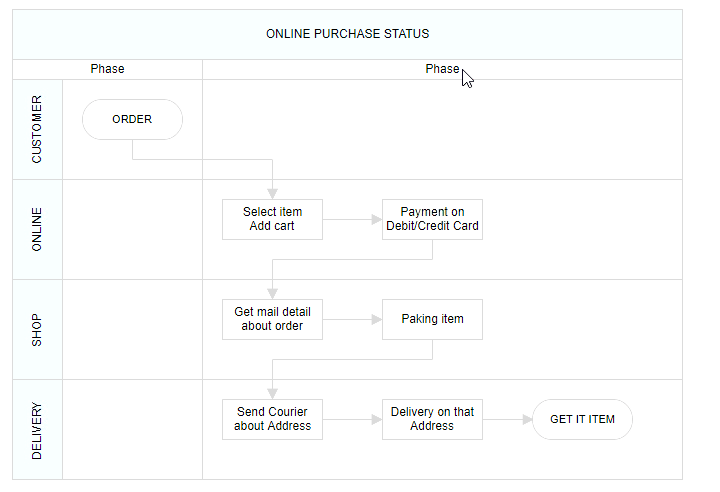## Lanes

Lane is a functional unit or a responsible department of a business process that helps to map a process within the functional unit or in between other functional units.

The number of `lanes` can be added to swimlane. The lanes are automatically stacked inside swimlane based on the order they are added.

### Create an empty lane

• The lanes `id` is used to define the name of the lane and its further used to find the lane at runtime and do any customization.

The following code example illustrates how to define a swimlane with lane.

emptylane.cs
``````using System;
using System.Collections.Generic;
using System.Linq;
using System.Web;
using System.Web.Mvc;
using Syncfusion.EJ2.Diagrams;
using System.Drawing;

namespace EJ2MVCSampleBrowser.Controllers.Diagram {
public partial class DiagramController: Controller {
// GET: Nodes
public ActionResult Nodes() {
List < DiagramNode > nodes = new List < DiagramNode > ();
List < DiagramNodeAnnotation > Node1 = new List < DiagramNodeAnnotation > ();
Content = "node1", Style = new DiagramTextStyle() {
Color = "White", StrokeColor = "None"
}
});
List<Lane> Lanes = new List<Lane>();
List<Phase> Phases = new List<Phase>();
swimlane.Shape  =  new SwimLane(){
Type = "SwimLane",
PhaseSize = 20,
{
Annotation = new DiagramNodeAnnotation() { Content = "ONLINE PURCHASE STATUS" },
Height = 50,
Orientation = "Horizontal",
Style = new DiagramTextStyle() { FontSize = 11 ,fill ="red"}
},
Lanes = Lanes,
Phases = Phases
};
ViewBag.nodes = nodes;
return View();
}
}
public class Node: DiagramNode {
public string text;
}
}``````

• The `header` property of lane allows you to textually describe the lane and to customize the appearance of the description.

The following code example illustrates how to define a lane header.

``````using System;
using System.Collections.Generic;
using System.Linq;
using System.Web;
using System.Web.Mvc;
using Syncfusion.EJ2.Diagrams;
using System.Drawing;

namespace EJ2MVCSampleBrowser.Controllers.Diagram {
public partial class DiagramController: Controller {
// GET: Nodes
public ActionResult Nodes() {
List < DiagramNode > nodes = new List < DiagramNode > ();
List < DiagramNodeAnnotation > Node1 = new List < DiagramNodeAnnotation > ();
Content = "node1", Style = new DiagramTextStyle() {
Color = "White", StrokeColor = "None"
}
});
List<Lane> Lanes = new List<Lane>();
{
Id = "stackCanvas1",
Height = 100,
{
Annotation = new DiagramNodeAnnotation() { Content = "Consumer" },
Width = 50,
},
});
List<Phase> Phases = new List<Phase>();
swimlane.Shape  =  new SwimLane(){
Type = "SwimLane",
PhaseSize = 20,
{
Annotation = new DiagramNodeAnnotation() { Content = "ONLINE PURCHASE STATUS" },
Height = 50,
Orientation = "Horizontal",
Style = new DiagramTextStyle() { FontSize = 11 ,fill ="red"}
},
Lanes = Lanes,
Phases = Phases
};
ViewBag.nodes = nodes;
return View();
}
}
public class Node: DiagramNode {
public string text;
}
}``````

The following code example illustrates how to customize the lane header.

``````using System;
using System.Collections.Generic;
using System.Linq;
using System.Web;
using System.Web.Mvc;
using Syncfusion.EJ2.Diagrams;
using System.Drawing;

namespace EJ2MVCSampleBrowser.Controllers.Diagram {
public partial class DiagramController: Controller {
// GET: Nodes
public ActionResult Nodes() {
List < DiagramNode > nodes = new List < DiagramNode > ();
List < DiagramNodeAnnotation > Node1 = new List < DiagramNodeAnnotation > ();
Content = "node1", Style = new DiagramTextStyle() {
Color = "White", StrokeColor = "None"
}
});
List<Lane> Lanes = new List<Lane>();
{
Id = "stackCanvas1",
Height = 100,
{
Annotation = new DiagramNodeAnnotation() { Content = "Consumer" },
Width = 50,Style = new DiagramTextStyle() { FontSize = 11 ,fill ="red"}
},
});
List<Phase> Phases = new List<Phase>();
swimlane.Shape  =  new SwimLane(){
Type = "SwimLane",
PhaseSize = 20,
{
Annotation = new DiagramNodeAnnotation() { Content = "ONLINE PURCHASE STATUS" },
Height = 50,
Orientation = "Horizontal",
Style = new DiagramTextStyle() { FontSize = 11 ,fill ="red"}
},
Lanes = Lanes,
Phases = Phases
};
ViewBag.nodes = nodes;
return View();
}
}
public class Node: DiagramNode {
public string text;
}
}``````

### Dynamic customization of lane header

You can customize the lane header style and text properties dynamically. The following code illustrates how to dynamically customize the lane header..

``````let lane : nodeModel = diagram.nodes;
diagram.dataBind();``````

You can add the a lane at runtime by using the client side API method called `addLanes`.

To add nodes to lane,you should add `children` collection of the lane.

The following code example illustrates how to add nodes to lane.

### Dynamically add children to lane

The diagram provides support to add children dynamically. The following code illustrates how to add children to lane dynamically.

`````` let node ={
id: 'Order',
shape: { type: 'Path', data: pathData },
annotations: [
{
content: 'ORDER',
style: { fontSize: 11 }
} ],
margin: { left: 60, top: 20 },
height: 40, width: 100
}

let lane : nodeModel = diagram.nodes;
swimlane.shape.lanes.childern=node;
diagram.dataBind();``````

### Resizing lane

• Lane can be resized in the bottom and left direction.
• Lane can be resized by using resize selector of the lane.
• Once you can resize the lane,the swimlane will be resized automatically.
• The lane can be resized either resizing the selector or the tight bounds of the child object. If the child node move to edge of the lane it can be automatically resized. The following image illustrates how resize the lane.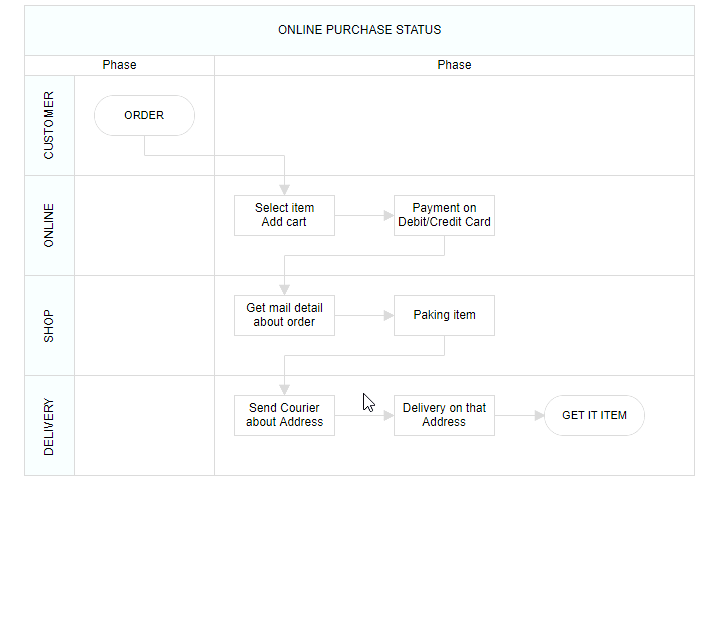### Lane swapping

• Lanes can be swapped using drag the lanes over another lane.
• Helper should intimate the insertion point while lane swapping. The following image illustrates how swapping the lane.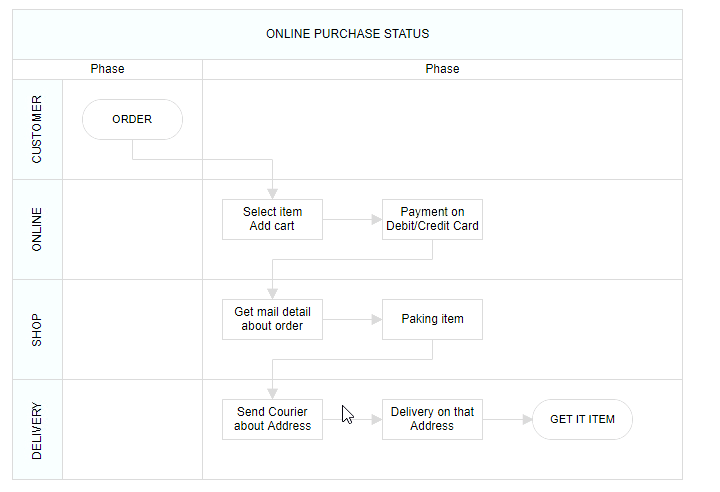### Disable Swimlane Lane swapping

You can disable swimlane lane swapping by using the property called `canMove`.

The following code illustrates how to disable swimlane lane swapping.

`````` let node ={
id: 'Order',
shape: { type: 'Path', data: pathData },
annotations: [
{
content: 'ORDER',
style: { fontSize: 11 }
} ],
margin: { left: 60, top: 20 },
height: 40, width: 100,canMove: false
}

let lane : nodeModel = diagram.nodes;
swimlane.shape.lanes.childern=node;
diagram.dataBind();``````

### Resize helper

• The special resize helper will be used to resize the lanes.
• The resize cursor will be available on the left and bottom direction alone.
• Once resize the lane the swimlane will be resized automatically.

### Children interaction in lanes

• You can resize the child node within swimlanes.
• You can drag the child nodes within lane.
• Interchange the child nodes from one lane to another lane.
• Drag and drop the child nodes from lane to diagram.
• Drag and drop the child nodes from diagram to lane.
• Based on the child node interactions,the lane size should be updated. The following image illustrates children interaction in lane.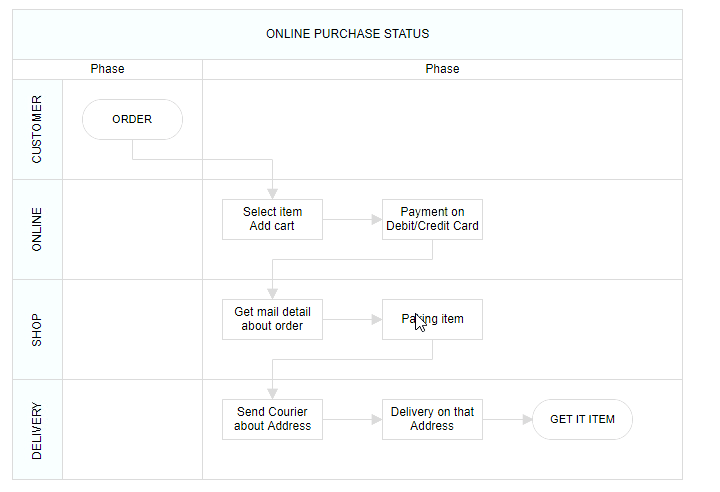Diagram provides the support to edit Lane headers at runtime. We achieve the header editing by double click event. Double clicking the header label will enables the editing of that. The following image illustrates how to edit the swimlane header.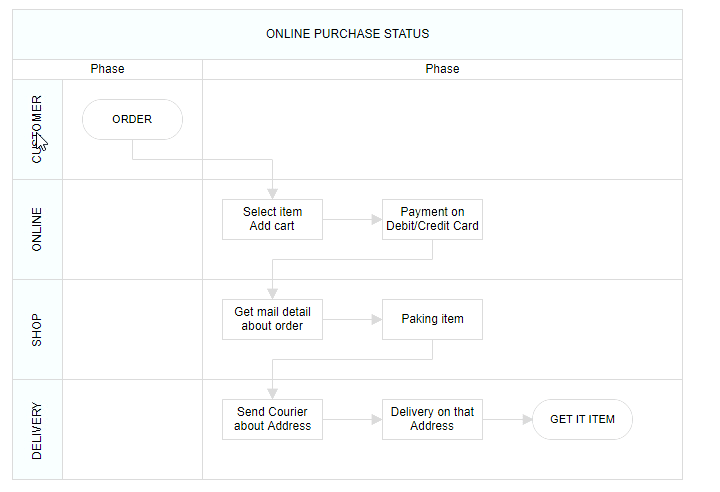## Phase

Phase are the subprocess which will split each lane as horizontally or vertically based on the swimlane orientation. The multiple number of `Phase` can be added to swimlane. The following code example illustrates how to add phase at swimlane.

phase.cs
``````using System;
using System.Collections.Generic;
using System.Linq;
using System.Web;
using System.Web.Mvc;
using Syncfusion.EJ2.Diagrams;
using System.Drawing;

namespace EJ2MVCSampleBrowser.Controllers.Diagram {
public partial class DiagramController: Controller {
// GET: Nodes
public ActionResult Nodes() {
List < DiagramNode > nodes = new List < DiagramNode > ();
List < DiagramNodeAnnotation > Node1 = new List < DiagramNodeAnnotation > ();
Content = "node1", Style = new DiagramTextStyle() {
Color = "White", StrokeColor = "None"
}
});
List<Lane> Lanes = new List<Lane>();
{
Id = "stackCanvas1",
Height = 100,
{
Annotation = new DiagramNodeAnnotation() { Content = "Consumer" },
Width = 50,Style = new DiagramTextStyle() { FontSize = 11 ,fill ="red"}
},
});
List<Phase> Phases = new List<Phase>();
{
Id= "phase1",
Offset=170,
{
Annotation = new DiagramNodeAnnotation() { Content = "Phase" },
},
});
swimlane.Shape  =  new SwimLane(){
Type = "SwimLane",
PhaseSize = 20,
{
Annotation = new DiagramNodeAnnotation() { Content = "ONLINE PURCHASE STATUS" },
Height = 50,
Orientation = "Horizontal",
Style = new DiagramTextStyle() { FontSize = 11 ,fill ="red"}
},
Lanes = Lanes,
Phases = Phases
};
ViewBag.nodes = nodes;
return View();
}
}
public class Node: DiagramNode {
public string text;
}
}``````

### Dynamically add phase to lane

You can add the a phase at runtime by using client side API method called `addPhases`. The following code example illustrates how to add phase at run time.

``````  let phase = [{
id: 'phase1', offset: 170,
header: { content: { content: 'Phase' } }
}]

### Customizing phase

The following code example illustrates how to customize the phase in swimlane.

phase.cs
``````using System;
using System.Collections.Generic;
using System.Linq;
using System.Web;
using System.Web.Mvc;
using Syncfusion.EJ2.Diagrams;
using System.Drawing;

namespace EJ2MVCSampleBrowser.Controllers.Diagram {
public partial class DiagramController: Controller {
// GET: Nodes
public ActionResult Nodes() {
List < DiagramNode > nodes = new List < DiagramNode > ();
List < DiagramNodeAnnotation > Node1 = new List < DiagramNodeAnnotation > ();
Content = "node1", Style = new DiagramTextStyle() {
Color = "White", StrokeColor = "None"
}
});
List<Lane> Lanes = new List<Lane>();
{
Id = "stackCanvas1",
Height = 100,
{
Annotation = new DiagramNodeAnnotation() { Content = "Consumer" },
Width = 50,Style = new DiagramTextStyle() { FontSize = 11 ,fill ="red"}
},
});
List<Phase> Phases = new List<Phase>();
{
Id= "phase1",
Offset=170,
{
Annotation = new DiagramNodeAnnotation() { Content = "Phase" },
},
});
swimlane.Shape  =  new SwimLane(){
Type = "SwimLane",
PhaseSize = 20,
{
Annotation = new DiagramNodeAnnotation() { Content = "ONLINE PURCHASE STATUS" },
Height = 50,
Orientation = "Horizontal",
Style = new DiagramTextStyle() { FontSize = 11 ,fill ="red"}
},
Lanes = Lanes,
Phases = Phases
};
ViewBag.nodes = nodes;
return View();
}
}
public class Node: DiagramNode {
public string text;
}
}``````

### Resizing

• The phase can be resized by using its selector.
• You must select the phase header to enable the phase selection.
• Once the phase can be resized, the lane size will be updated automatically.

### Resizing helper

• The special resize selector will be used to resize the phase.
• The resize cursor will be available on the left and bottom direction for horizontal, and the top and bottom direction for vertical swimlane.

Diagram provides the support to edit phase headers at runtime. We achieve the header editing by double click event. Double clicking the header label will enables the editing of that. The following image illustrates how to edit the phase header.Diagram provides support to add swimlane and phases to symbol palette. The following code sample illustrate how to add swimlane and phases to palette.

The following code example illustrates how to customize the phase in swimlane.

node.cs
``````using System;
using System.Collections.Generic;
using System.Linq;
using System.Web;
using System.Web.Mvc;
using Syncfusion.EJ2.Diagrams;
using System.Drawing;

namespace EJ2MVCSampleBrowser.Controllers.Diagram {
public partial class DiagramController: Controller {
// GET: Nodes
public ActionResult Nodes() {
List < DiagramNode > nodes = new List < DiagramNode > ();
List < DiagramNodeAnnotation > Node1 = new List < DiagramNodeAnnotation > ();
Content = "node1", Style = new DiagramTextStyle() {
Color = "White", StrokeColor = "None"
}
});
Id = "node1",
Width = 100,
Height = 100,
BorderWidth=2,
Style = new NodeStyleNodes() {
Fill = "darkcyan"
},
text = "node1",
OffsetX = 100,
OffsetY = 100,
Annotations = Node1
});
ViewBag.nodes = nodes;

return View();
}
}
public class Node: DiagramNode {
public string text;
}
}``````

### Drag and drop swimlane to palette

• The drag and drop support for swimlane shapes has been provided.
• When you drag and drop the lane shape,if the diagram already contains swimlane with the same orientation, the lane will be added and stacked inside a swimlane based on the order. Otherwise, it will be added a new swimlane.
• The phase will only drop on swimlane shape with same orientation.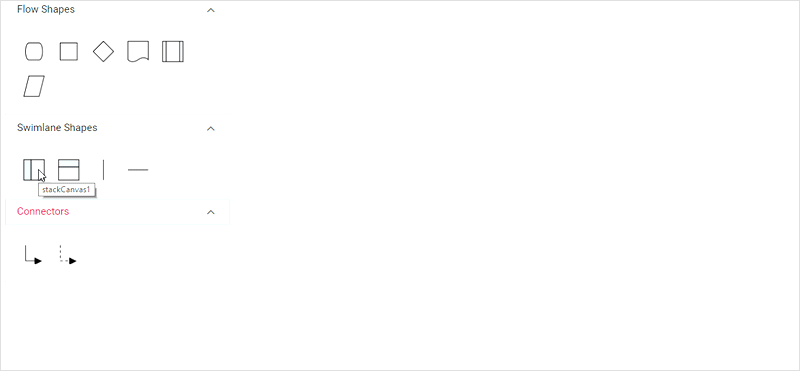## Limitations

• Connectors cannot be canceled when added directly to swimlane. You must initialize the connector through connector collection.
• We cannot edit the phase line style.